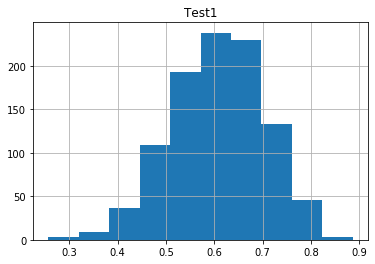Histograms are the backbone to understanding distribution within your series of data. Pandas Histogram provides an easy way to plot a chart right from your data.

Histogram plots traditionally only need one dimension of data. It is meant to show the count of values or buckets of values within your series.

Pandas` DataFrame.hist()` will take your DataFrame and output a histogram plot that shows the distribution of values within your series. The default values will get you started, but there are a ton of customization abilities available.

There are multiple ways to make a histogram plot in pandas. We are going to mainly focus on the first

```1. pd.DataFrame.hist(column='your_data_column')
2. pd.DataFrame.plot(kind='hist')
3. pd.DataFrame.plot.hist()```

This function is heavily used when displaying large amounts of data. Pandas will show you one histogram per column that you pass to .hist()

## Pandas Histogram

The default `.histogram()` function will take care of most of your needs. However, the real magic starts to happen when you customize the parameters. Specifically the `bins` parameter.

`Bins` are the buckets that your histogram will be grouped by. On the back end, Pandas will group your data into bins, or buckets. Then pandas will count how many values fell into that bucket, and plot the result.

Another way to describe `bins`, how many bars do you want in your histogram chart? A lot or a little?

### Histogram Parameters

Before we get into the histogram specific parameters, keep in mind that Pandas charts inherit other parameters from the general Pandas Plot function. These other parameters will deal with general chart formatting vs scatter specific attributes. We recommend viewing these for full chart flexibility. We’ll use some in our example below.

• column: This is the specific column(s) that you want to call histogram on. By default, pandas will create a chart for every series you have in your dataset.
• by: This parameter will split your data into different groups and make a chart for each of them. Check out the example below where we split on another column.
• bins (Either a scalar or a list): The number of bars you’d like to have in your chart. Or another way, the number of buckets you would like to group your data into. If you pass a list instead of a scale, Pandas will make bins with edges of your list values.
• formatting parameters: There are a bunch of other formatting parameters that will help you customize the look of your chart. I encourage your to check them out on the official pandas hist page.

Let’s look at a fun example

In :
```import pandas as pd
import numpy as np
import matplotlib.pyplot as plt
```

### Pandas Histogram¶

Not only can Pandas handle your data, it can also help with visualizations. Let's run through some examples of histogram. We will be using the San Francisco Tree Dataset. To download the data, click "Export" in the top right, and download the plain CSV. Or simply clone this repo.

Examples:

1. Default Histogram plot
2. Histogram plot w/ 30 bins
3. Histogram plot w/ custom bins
4. Plotting multiple groups
5. Extra customized scatter plot using the general DataFrame.plot() and .hist() parameters
6. Using all the parameters, but plotting multiple Series

First, let's import our data

In :
```np.random.seed(seed=42)
data_points = 1000

df = pd.DataFrame(data=list(zip(np.random.choice(["Math", "English"], size=data_points),
np.random.beta(15, 10, size=data_points),
np.random.beta(30, 4, size=data_points))),
columns=['Major', 'Test1', 'Test2'])

```
Out:
MajorTest1Test2
0Math0.4887520.778087
1English0.5046090.894973
2Math0.3805670.864508
3Math0.7036820.846423
4Math0.3836400.904955

### 1. Default Histogram Plot¶

In order to create a histogram in pandas, all you need to do is tell pandas which column you would like to supply the data. In this case, I'm going to tell pandas I want to see the distribution of scores (histogram) for Test 1.

As you can see, this 1-liner produces a chart for you on your data.

In :
```df.hist(column='Test1');
```### 2. Histogram plot w/ 30 bins¶

The easy way to think about bins is "how many bars do you want in your bar chart?" The more bins, the higher resolution your data. Picking the number of bins is both an art and a science. Play around with a few values and see what you like.

In this case, 2 bins doesn't tell me much, 200 is too many, but 35 feels nice.

In :
```df.hist(column='Test1', bins=2);
```In :
```df.hist(column='Test1', bins=200);
```In :
```df.hist(column='Test1', bins=35);
```### 3. Histogram plot w/ custom bins¶

Notice how your bins are evenly spaced out for you in the charts above? Well you could also create your own bins. You'd generally do this when you want buckets of different sizes. Here I'm going to create 3 buckets: test scores <.5, .5-.75 and .75+.

Over 500 students got a test score less than .5 -- interesting!

In :
```df.hist(column='Test1', bins=[0,.5,.75,1]);
```### 4. Plotting multiple groups¶

You can also plot multiple groups side by side. Here I want to see two histograms, one of the english majors and one of the math majors. You do this by setting the 'by' parameter.

In :
```df.hist(column='Test1', by='Major')
```
Out:
```array([<matplotlib.axes._subplots.AxesSubplot object at 0x7fc2a91d4c50>,
<matplotlib.axes._subplots.AxesSubplot object at 0x7fc2a59976d0>],
dtype=object)```### 5. Extra customized scatter plot using the general DataFrame.plot() and .hist() parameters¶

Next up, let's customize our chart a bit more. I'm going to use extra parameters from .hist() and a few general parameters from matplotlib plot

In :
```df.hist(column='Test1',
grid=False,
figsize=(10, 4),
legend=True,
bins=30,
orientation='horizontal',
color='#FFCF56');
```### 6. Using all the parameters, but plotting multiple Series¶

To plot multiple series, I like to use the df.plot(kind='hist) method. It's an easier one liner to use.

In :
```df.plot(kind='hist',
alpha=0.7,
bins=30,
title='Histogram Of Test Scores',
rot=45,
grid=True,
figsize=(12,8),
fontsize=15,
color=['#A0E8AF', '#FFCF56'])
plt.xlabel('Test Score')
plt.ylabel("Number Of Students");
```